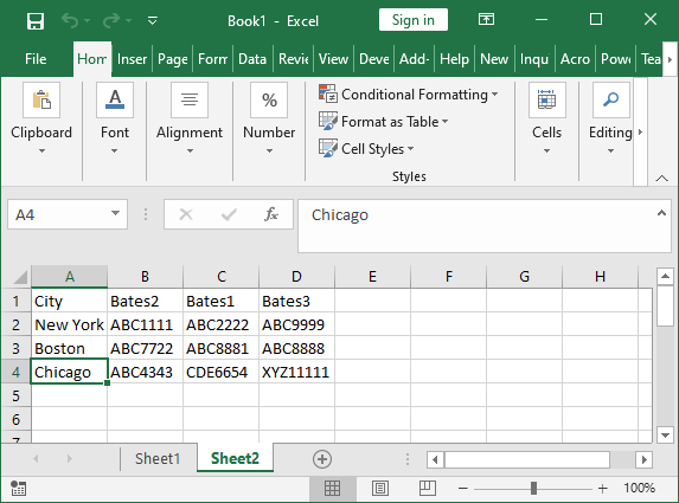top of pageSearch

# Sorting by Rows in Excel

You can use the below vba code, posted here, to sort data on an Excel worksheet by rows instead of columns.

As currently written, this macro will sort from row 2 to the end. To clear, in this example, what happens is it sorts the entries in each row from 2 to 4, so the data in B2 through D2 is in alpha order from left to right; then from B3 through D3 from left to right; and so on.

We begin with:. . . and get:Sub sortrows()

Dim i As Long Dim lr As Long

lr = Range("A" & Rows.Count).End(xlUp).Row For i = 2 To lr Range("B" & i, "D" & i).Sort Key1:=Range("B" & i), _ Order1:=xlAscending, _ Header:=xlNo, _ OrderCustom:=1, _ MatchCase:=False, _ Orientation:=xlLeftToRight, _ DataOption1:=xlSortNormal Next i

End Sub

bottom of page